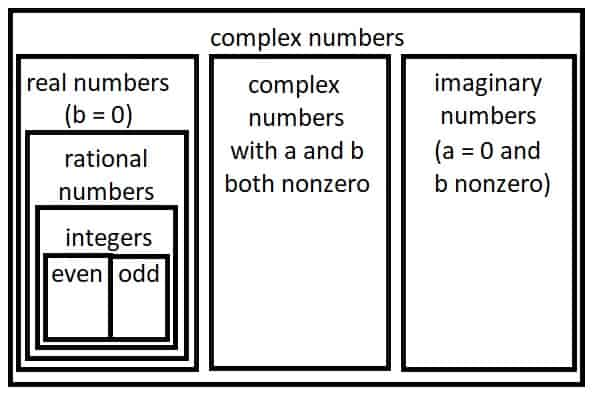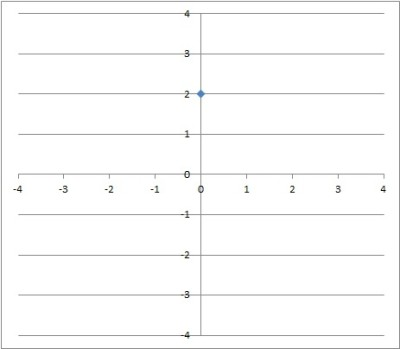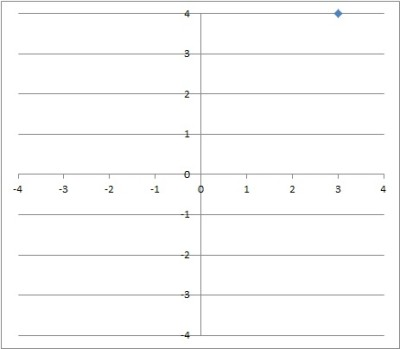# What Is A Pure Imaginary Number? (3 Key Ideas To Know)

Imaginary numbers are useful throughout mathematics, including algebra (for solving some quadratic equations) calculus, and differential equations.

So, what is a pure imaginary number?  A pure imaginary number is a complex number whose real part is zero (that is, Re(z) = 0). So, if a complex number z has the form a + bi and a = 0, then z = bi is a pure imaginary number. A pure imaginary number bi is a square root of the negative number –b2 (the other square root of –b2 is –bi).

Of course, the word “pure” is not necessary – a pure imaginary number is the same as an imaginary number, since both have a real part that is zero.

In this article, we’ll talk about (pure) imaginary numbers and answer some questions about them.  We’ll also take a look at how to graph imaginary and complex numbers.

Let’s get started.

## What Is A Pure Imaginary Number?

A pure imaginary number is a specific type of complex number.  A complex number a + bi is the sum of a real part “a” and an imaginary part “bi”.An imaginary number is a specific type of complex number – one where the real part is zero (a = 0).

A pure imaginary number has a real part that is zero – that is, a = 0.  So, a pure imaginary number has the form 0 + bi, or just bi.

Another notation you may see is Re(z) = 0.  Re(z) just means “the real part of the complex number z”.  So Re(z) = 0 means that the real part of the complex number z is zero.

A pure imaginary number is one square root of a negative number (the other square root is the negative of that number).

For example, 2i is a square root of -4, and -2i is another square root of -4, since:

• (2i)2 = 4i2 = 4(-1) = -4
• (-2i)2 = 4i2 = 4(-1) = -4

### How Imaginary Numbers Work

Imaginary numbers work by giving us a way to talk about the “square root of a negative number”.  We start out by defining the imaginary unit i = √-1.

So, i is the square root of -1, and so i2 = -1.  We can also say that i3 = -i and i4 = 1, since:

• i3 = i2*i = (-1)*i = -i
• i4 = i2*i2 = (-1)*(-1) = 1

We can use these four basic powers of i (along with the rules of exponents) to simplify any power of i.  For example:

• i2022 = (i2)1011 = (-1)1011 = -1 (since 1011 is odd)

Once we have defined the imaginary unit i = √-1, we can use it to define other imaginary numbers.  For example:

• √-4 = √4√-1 = 2i

Note that -2i is also a square root of -4.

More generally, the two square roots of a negative number –b2 are bi and –bi.

So, the set of imaginary number is the set:

• {bi | b is a real number}

We can also write this as:

• {a + bi | a = 0  and b is a real number}

This is a subset of the set of complex numbers, which are defined by:

• {a + bi | a and b are real numbers}

So, a complex number is just a sum of a real number (the “a” part) and an imaginary number (the “bi” part).

### What Is The Square Root Of The Imaginary Unit? (Square Root Of i)

First, remember that the square root of i is a complex number, so it has the form a + bi.

So, we want a number a + bi such that:

• (a + bi)*(a + bi) = i

First, let’s use FOIL on the left side, then we will combine like terms and use the powers of i:

• a2 + abi + abi + b2i2 =i  [used FOIL on the left side]
• a2 + 2abi + b2i2 = i  [combined like terms]
• a2 + 2abi + b2(-1) = i  [used i2 = -1]
• (a2 – b2) + (2ab)I = 0 + 1i  [grouped real and imaginary parts]

When we set the real terms on both sides of the equation equal to each other, we get:

• a2 – b2 = 0

This implies that (a + b)(a – b) = 0  [factored by a difference of squares!]

So a + b = 0, or a – b = 0, meaning a = -b, or a = b.

Now, when we set the imaginary terms on both sides of the equation equal to each other, we get:

• 2abi = 1i
• 2ab = 1
• ab = 1/2

Combining this with the equations above, we get two possibilities.

For a = -b:

• ab = 1/2
• -b*b = 1/2
• -b2 = 1/2
• b2 = -1/2

This is impossible, since b is a real number (not imaginary).

For a = b:

• ab = 1/2
• b*b = 1/2
• b2 = 1/2
• b = √(1/2)
• b = √2 / 2

Since a = b, this means a = √2 / 2 also.  So one of the square roots of i is:

• a + bi = (√2 / 2) + (√2 / 2)i = (√2 / 2)(1 + i)

The negative of this number is the other square root of i:

• (-√2 / 2) – (√2 / 2)i = (√2 / 2)(1 – i)

### Is Zero A Pure Imaginary Number?

Zero is a pure imaginary number, since its real part is zero.  That is, we can write the number zero as follows:

• 0 = 0 + 0i

which has the form a + bi, meaning 0 = 0 + 0i is a complex number (a = 0, b = 0).  The real part is equal to zero (a = 0), so 0 = 0 + 0i is a pure imaginary number.

Note that zero is also a real number, since its imaginary part is zero (b = 0).

### Can Imaginary Numbers Be Negative?

Imaginary numbers cannot be negative.  The concepts of positive and negative only apply to nonzero real numbers.

Remember that:

• a positive number is to the right of zero on the real number line
• a negative number is to the left of zero on the real number linePositive numbers (blue) are to the right of zero on the real number line, while negative numbers (red) are to the left of zero on the real number line. Imaginary numbers are not on the real number line, so they cannot be positive or negative.

On the other hand, an imaginary number is not on the real number line.  So, we cannot say which side of zero an imaginary number can be found.

Thus, an imaginary number is neither positive nor negative.

However, we can take the coefficient of the imaginary unit i and look at its sign.  That is, in the imaginary number bi, we can tell if the value of b is positive or negative.

For example:

• 2i is an imaginary number that is neither positive nor negative.  However, b = 2 is positive.
• -5i is an imaginary number that is neither positive nor negative.  However, b = -5 is negative.

### Can Imaginary Numbers Be Rational?

Imaginary numbers cannot be rational, since a rational number is a real number, and an imaginary number is not a real number.

However, we can take the coefficient of the imaginary unit i and determine if it is rational.  That is, in the imaginary number bi, we can tell if the value of b is rational or not.

For example:

• 0.4i is an imaginary number that is not rational.  However, b = 0.4 can be written as the fraction 2/5, which means b is rational.
• (√2)*i is an imaginary number that is not rational.  However, b = √2 cannot be written as a ratio of two integers, so it is irrational.

### Can Imaginary Numbers Be Irrational?

Imaginary numbers cannot be irrational, since an irrational number is a real number, and an imaginary number is not a real number.

However, we can take the coefficient of the imaginary unit i and determine if it is irrational or not (see the examples above).  That is, in the imaginary number bi, we can tell if the value of b is irrational or not.

### Can You Have An Imaginary Number In The Denominator?

You can have an imaginary number in the denominator of a fraction.  This is a perfectly valid expression.

#### Example 1: A Fraction With An Imaginary Number In The Denominator

Let’s say we have the fraction 1 / i.  This is a fraction with an imaginary denominator.

We can simplify this fraction as follows:

• 1 / i
• =(1 / i)*(i / i)  [multiply by i / i]
• = 1*i / i*i
• =i / i2
• =i / -1  [since i2 = -1]
• =-i

So 1 / i is equal to –i (which is also equal to i3).

#### Example 2: A Fraction With A Complex Number In The Denominator

Let’s say we have the fraction 1 / (2 + i).  This is a fraction with a complex denominator.

We can simplify this fraction as follows:

• 1 / (2 + i)
• =(1 / (2 + i)*((2 – i) / (2 – i))  [multiply by (2 – i) / (2 – i), since 2 – i is the complex conjugate of 2 + i]
• = 1*(2 – i) / (2 + i)*(2 – i)
• =(2 – i) / (4 + 2i – 2i – i2)  [FOIL the denominator]
• =(2 – i) / (4 – i2)  [combine like terms in the denominator]
• =(2 – i) / (4 – (-1))  [since i2 = -1]
• =(2 – i) / 5

So 1 / (2 + i) is equal to (2 – i) / 5 or (2/5) – (2/5)i.

### Can You Square An Imaginary Number?

You can square an imaginary number, and the result will always be a negative number (or zero when the imaginary number is zero).  We can prove this as follows.

Let bi be an imaginary number (so b is a real number).

When we square bi, we get:

• (bi)2
• =b2i2
• =b2(-1)  [since i2 = -1]
• =-b2

Since b is real, then b2 >= 0, and so –b2 <= 0.  That is, (bi)2 is negative for nonzero b, and zero for b = 0.

### Can Imaginary Numbers Be Graphed?

You can graph imaginary numbers.  However, you need to use a real axis (x-axis) and an imaginary axis (y-axis) to graph complex or imaginary numbers.

Here are some examples.

#### Example 1: Graphing The Imaginary Number 2i

The number 2i is really 0 + 2i, which is a complex number of the form a + bi (a = 0, b = 2).  So, we would graph this as the point where a = 0 (real axis) and b = 2 (imaginary axis).

This point is 2 units above the origin in the complex plane.  You can see this illustrated below.You can see the imaginary number 2i (0 + 2i) graphed in the complex plane (it is the blue dot on the y axis, 2 units above the origin).

#### Example 1: Graphing The Complex Number 3 + 4i

The number 3 + 4i is really a complex number of the form a + bi (a = 3, b = 4).  So, we would graph this as the point where a = 3 (real axis) and b = 4 (imaginary axis).

This point is 5 units away from the origin in the complex plane.  You can see this illustrated below.You can see the complex number 3 + 4i graphed in the complex plane (it is the blue dot 3 units right and 4 units above the origin).

## Conclusion

Now you know what a pure imaginary number is and how they are connected to square roots of negative numbers.

You can learn all about the uses of imaginary numbers here.